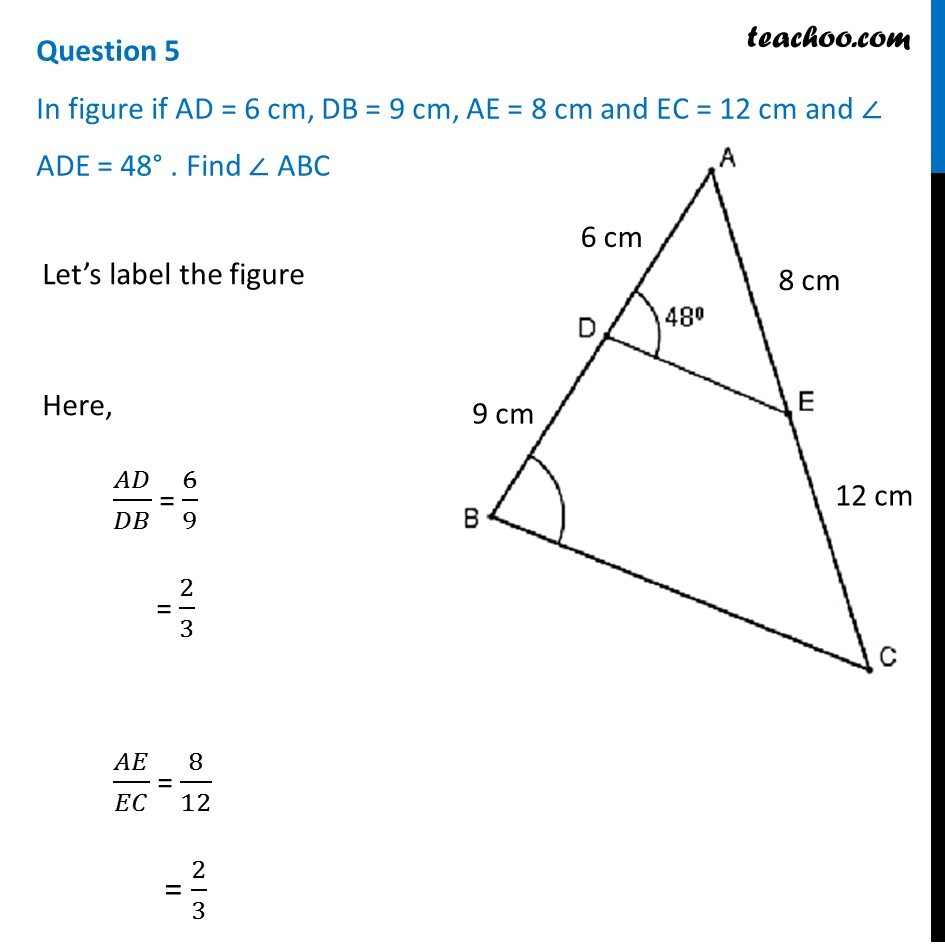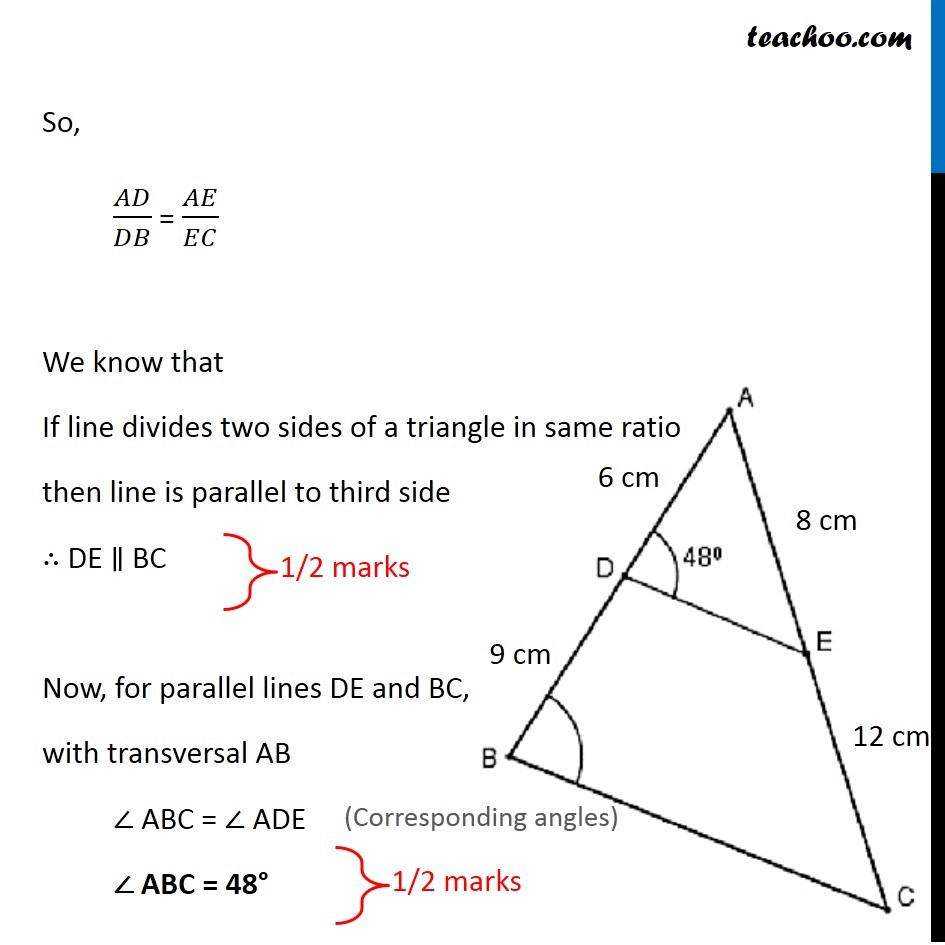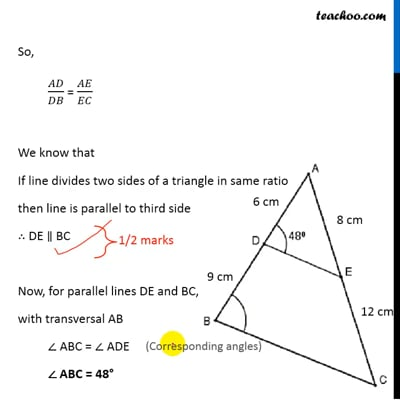CBSE Class 10 Sample Paper for 2019 Boards

Class 10
Solutions of Sample Papers for Class 10 Boards

Question 5

In figure if AD = 6 cm, DB = 9 cm, AE = 8 cm and EC = 12 cm and ∠ ADE = 48° . Find ∠ ABCThis video is only available for Teachoo black users

Learn in your speed, with individual attention - Teachoo Maths 1-on-1 Class

### Transcript

Question 5 In figure if AD = 6 cm, DB = 9 cm, AE = 8 cm and EC = 12 cm and ∠ ADE = 48° . Find ∠ ABC Let’s label the figure Here, 𝐴𝐷/𝐷𝐵 = 6/9 = 2/3 𝐴𝐸/𝐸𝐶 = 8/12 = 2/3 So, 𝐴𝐷/𝐷𝐵 = 𝐴𝐸/𝐸𝐶 We know that If line divides two sides of a triangle in same ratio then line is parallel to third side ∴ DE ∥ BC Now, for parallel lines DE and BC, with transversal AB ∠ ABC = ∠ ADE ∠ ABC = 48°